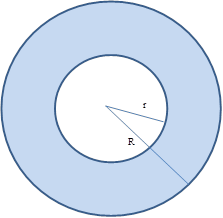Chapter 8.4, Problem 31EElementary Geometry For College St...

7th Edition
Alexander + 2 others
ISBN: 9781337614085

Solutions

Chapter
SectionElementary Geometry For College St...

7th Edition
Alexander + 2 others
ISBN: 9781337614085
Textbook Problem

Given concentric circles with radii of lengths R and r, where R > r , explain why A r i n g = π ( r + r ) ( R − r ) .To determine

To explain:

Aring=π(R+r)(Rr), for concentric circles with the radii of lengths R and r.

Explanation

Two circles with a common center are called concentric circles.

A region bounded by two concentric circles is called ring.

Calculation:

The length of the radius of the outer circle is R.

The area of the outer circle is πR2.

Next, the length of the radius of the inner circle is r.

The area of the inner circle is πr2.

Now to find the area of the ring, subtract the area of the inner circle from the area of the outer circle.

Therefore, Aring=πR2πr2

Still sussing out bartleby?

Check out a sample textbook solution.

See a sample solution

The Solution to Your Study Problems

Bartleby provides explanations to thousands of textbook problems written by our experts, many with advanced degrees!

Get Started

Solve the equations in Exercises 112 for x (mentally, if possible). x1=cx+d(c1)

Finite Mathematics and Applied Calculus (MindTap Course List)

Evaluate the integral. 03(2sinxex)dx

Single Variable Calculus: Early Transcendentals, Volume I

Find the derivatives of the functions in Problems 1-34. 29.

Mathematical Applications for the Management, Life, and Social Sciences

Evaluate each expression: 83(42)

Elementary Technical Mathematics

Find the area of the shaded region. 8.

Single Variable Calculus: Early Transcendentals

True or False: f(x) = 3x x3 is concave down for x 1.

Study Guide for Stewart's Single Variable Calculus: Early Transcendentals, 8th